SSAT Elementary Level Math : How to find the whole from the part

Example Questions

Example Question #41 : Describing And Comparing Measurable Attributes

Which line is shorter?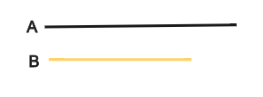Line B

Line A

They are the same length

Line B

Explanation:

Line B is shorter than line A.

Example Question #51 : Describing And Comparing Measurable Attributes

Which line is shorter?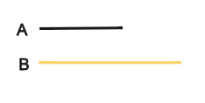Line B

Line A

They are the same length

Line A

Explanation:

Line A is shorter than line B.

Example Question #52 : Describing And Comparing Measurable Attributes

Which line is shorter?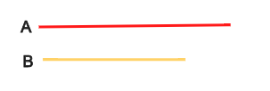Line A

Line B

They are the same length

Line B

Explanation:

Line B is shorter than line A.

Example Question #51 : Describing And Comparing Measurable Attributes

Which line is shorter?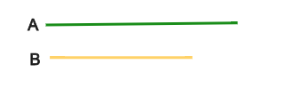They are the same length

Line A

Line B

Line B

Explanation:

Line B is shorter than line A.

Example Question #42 : Compare Two Objects With A Measurable Attribute: Ccss.Math.Content.K.Md.A.2

Which line is shorter?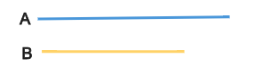They are the same length

Line A

Line B

Line B

Explanation:

Line B is shorter than line A.

Example Question #51 : Measurement & Data

Which line is shorter?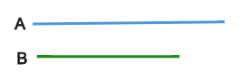Line B

They are the same length

Line A

Line B

Explanation:

Line B is shorter than line A.

Example Question #44 : Compare Two Objects With A Measurable Attribute: Ccss.Math.Content.K.Md.A.2

Which line is shorter?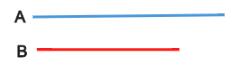They are the same length

Line B

Line A

Line B

Explanation:

Line B is shorter than line A.

Example Question #7 : Identifying Numbers

What number is ninety-six?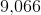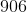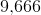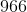Explanation:in word form is ninety-six.

Example Question #8 : Identifying Numbers

What number is nine hundred forty-eight?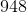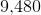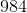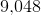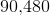Explanation:in word form is nine hundred forty-eight.

Example Question #91 : How To Find The Whole From The Part

Which statement is true?

There is no true statement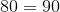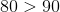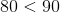The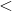sign means less than.is less thanbecause of the number in the tens place.has a lesser value than, sois less than